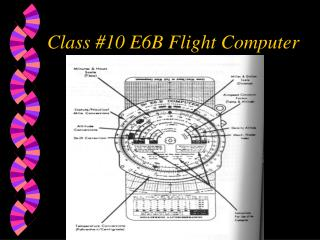Download PresentationClass #10 E6B Flight Computer

# Class #10 E6B Flight Computer

Download Presentation## Class #10 E6B Flight Computer

- - - - - - - - - - - - - - - - - - - - - - - - - - - E N D - - - - - - - - - - - - - - - - - - - - - - - - - - -
##### Presentation Transcript

1. Class #10 E6B Flight Computer

2. Content of lesson • Time-distance problems • Fuel consumption • Statute-to-nautical conversion • True Airspeed • True Altitude

3. HOMEWORK • JEPPESEN PRIVATE PILOT MANUAL CHAPTER 8 SECTION C • PHAK 15-11 TO 15-16 • NO ASA/GLEIM QUESTIONS ON THE NEXT TEST • HOWEVER STUDY CHAPTER 9 (ASA 9-14 to 9-36) and do homework

4. SLIDE RULE MATHEMATICS • MULTIPLIER OVER 10 (found on INNER SCALE) = PRODUCT OVER OTHER FACTOR • 2 x 4 = 8 • MULTIPLIER 2 (OVER 10 ON INSIDE SCALE) FIND #4 ON INNER SCALE AND ANSWER OR PRODUCT ON OUTERSCALE

5. SLIDE RULE MATH • DIVISION • DIVIDEND OVER DIVISOR = QUOTIENT (found OVER 10 on inner scale) • 8 / 4 = 2

6. PROBLEMS • 13 x 7 = • 300 / 15 = • 19 x 5 = • 345 / 15 = • 137 x 7 =

7. PROBLEMS • 13 x 7 = 91 • 300 / 15 = 20 • 19 x 5 = 95 • 345 / 15 = 23 • 137 x 7 = 959

8. Converting Minutes to Equivalent Hours

9. Velocity • Velocity = Distance / Time • Distance = Velocity X Time • Time = Distance / Velocity • When you use time make sure you convert the minutes to equivalent hours

10. E6B • Please note that the stationary scale is the main scale (distance) while the inner scale is denoting time.

11. Time, Ground Speed, Distance problems • On all Time, Ground Speed, Distance problems the large triangular index is either set opposite Ground Speed or will position itself opposite Ground Speed. • Distance and time are always opposite each other.

12. Time, Ground Speed, Distance problems

13. Time, Ground Speed, Distance problems • Given a speed of 120 knots, Distance of 200 N.M. what is the time? • 1:40 or 100 minutes • V=d/t (ground speed = distance / time • T=D/V = 200/120=1.67=1:40

14. Time, Ground Speed, Distance problems • Given a time 2 hours, Distance of 200 knots what is the speed? • 100 knots

15. Time, Ground Speed, Distance problems (4) • Speed = ?, Time=1:30, Distance=165 n.m. • Speed=132 mph, Time=?, Distance=198 mi • S=166mph, T=1:50, D=? • S=?, T=2:10, D=206 mi • S=190kts, T=?, D=95N.M • S=130MPH, T=1:10, D=? • S=180MPH, T=?, D=330

16. Fuel consumption problems • Fuel rate is read under the triangular index (usually gal/hr). • The outer scale is equivalent to the fuel used • The inner scale is flying time • GPH=#GALLONS / TIME • Calculate the same as time distance speed problems

17. Fuel Consumption Problems

18. Fuel Consumption Problems • Fuel Rate=13 gal. Per hour • Time=1:30 (90minutes) • Find: Total fuel:? • 19.5 gallons

19. Fuel Consumption Problems • FR= FUEL RATE, T=TIME, TF=TOTAL FUEL • FR=18, T=1:50, TF=? • FR=14, T=?, TF=21 • FR=? , T= 2:30, TF=25 • FR=13 , T=?, TF=40 • FR=6 , T=3:30 , TF=?

20. Statute to Nautical Mile conversion

21. True Airspeed

22. Density Altitude

23. True Airspeed &Density Altitude • Pressure Altitude, Temp., IAS • 8000ft, -10C, 120kts • 9000ft, +10C, 140mph • 5000ft, +30C, 120mph • 7000ft, -20C, 150 kts • 12000ft, -10C, 180mps

24. Answers • TAS, Density Altitude • 133 kts, 6900ft • 164 mph, 10,500ft • 135 mph, 8000ft • 160 kts, 4500ft • 216 mph, 12,000ft

25. Temperature Conversion Scale • Bottom of E6B • -10 C = what in F • -40 C = F • 30 C = ?

26. U.S. Gallons to Imperial Gallons • On APR Airlearn E6B • Outside Imperial gallons between 18 and 19 • Outside U.S. gallons near 22 • On ASA different

27. True and Absolute Altitude • Given: Pressure altitude 12,000ft • Indicated Altitude 11,500 • (OAT) -10C • Terrain Elevation 10,500 • What is the absolute altitude

28. True and Absolute Altitude P208

29. True and Absolute Altitude P208 • What is the absolute altitude • 500ft • 950ft • 1000ft • 1500ft • To figure out you need to figure out True altitude first

30. True and Absolute Altitude P208 • True Altitude = 11,450ft • Absolute altitude = 950 ft

31. Fuel Reserve BBCC/FAR’s • BBCC = 45 minutes day or night • FAR = 30 min day, 45 min night